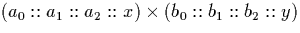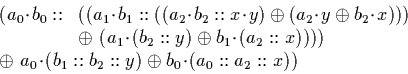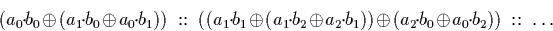Next: Signed Binary Stream Multiplication Up: Multiplication Previous: Multiplication

### Dyadic Stream Multiplication

Dyadic stream multiplication (section 4.2.2) requires (n+1) input digits to generate n digits of output. This is clear from the algorithm which computes the average of two streams. The first digit of the first of these is determined directly from the first digits of the input, but the first digit of the second stream comes from the second digits of the two input streams.

The dyadic multiplication algorithm branches. Although only one recursive call to the multiplication function is made for each new digit, two stream average operations are also required. This means that n output digits will result in (2n-2) average operations running concurrently. There is also the possibility of the size of the dyadic digits swelling as individual digits are multiplied at each step.

Consider. Using the algorithm we can expand this and get the first two digits as follows:Which gives us a result whose first two digits are as follows:As we proceed, there are more and more average operations have been performed for each digit of output. In most cases where the input digits are random, a large numerator and denominator will be required to represent the result.Next: Signed Binary Stream Multiplication Up: Multiplication Previous: Multiplication
Martin Escardo
5/11/2000